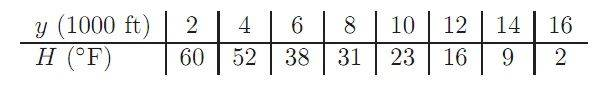# Solving a Related Rates Problem: Calculating Eva Wisnierska's Temperature Change

• oates151
In summary, the problem asks for the rate of change in ambient temperature (in ◦F/min) when paraglider Eva Wisnierska was at 4000 feet, given that she was ascending at a speed of 3000 ft/min and the temperature at various heights was recorded. The given data suggests that the temperature was decreasing at a rate of -5.5 deg F/1000 ft between 2000 ft and 6000 ft. However, using only the two surrounding points in the data would provide a more accurate estimate.

## Homework Statement

On February 16, 2007, paraglider Eva Wisnierska was caught in a freak thunderstorm over
Australia and carried upward at a speed of about 3000 ft/min. The table below gives the
temperature at various heights. Approximately how fast (in ◦F/min) was her ambient
temperature decreasing when she was at 4000 feet?## The Attempt at a Solution

Well here's where I've gotten.

I am not sure, but this problem looks like some sort of related rates problem. We known dy/dt is 3000 ft/min, but we need in deg F/min, so what I did is a simple slope calculation for the point 4000 ft by doing

(60-52)/(2-4) and getting -4 deg F/1000 ft

From here, I'm not exactly sure how to manipulate and get the rate that we need. Am I on the right track? What's next?

Thanks so much!

-Pat

oates151 said:

## Homework Statement

On February 16, 2007, paraglider Eva Wisnierska was caught in a freak thunderstorm over
Australia and carried upward at a speed of about 3000 ft/min. The table below gives the
temperature at various heights. Approximately how fast (in ◦F/min) was her ambient
temperature decreasing when she was at 4000 feet?## The Attempt at a Solution

Well here's where I've gotten.

I am not sure, but this problem looks like some sort of related rates problem. We known dy/dt is 3000 ft/min, but we need in deg F/min, so what I did is a simple slope calculation for the point 4000 ft by doing

(60-52)/(2-4) and getting -4 deg F/1000 ft

From here, I'm not exactly sure how to manipulate and get the rate that we need. Am I on the right track? What's next?
I would use the two alt., temp. points on either side. Between 2000' and 4000' the temperature dropped 8 deg F., but between 4000' and 6000', the temperature drop was quite a bit more. You would get a better estimate by using the two surrounding points in your data.

I disagree that this is a related rates problem. As I see it, it's a problem about estimating the slope of a curve from a set of data.

Oh, I see. So on that case, in order to get a more accurate method, i'd calculate the slope between the two surrounding points which gets us -5.5 deg f/1000 ft.

Now, they want the units in in deg F/min. Since she's carried upward at 3000 ft/min and the temperature is decreasing at -5.5 deg f/1000 ft, then

(3000 ft/min)(-5.5 deg f/1000ft)= 3 x -5.5 /min = 36 x -5.5 = -198 in deg F/min ?

No, I don't think so. Her vertical speed is 3000 ft/min. She rose from 2000' to 6000' in 4000/3000 minutes, or 4/3 minute, during which time the temperature dropped 22 deg. F. That's NOT going to be a drop of 198 deg F per minute.